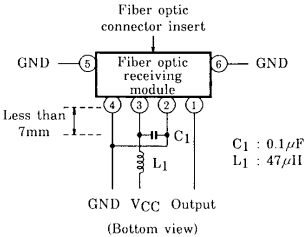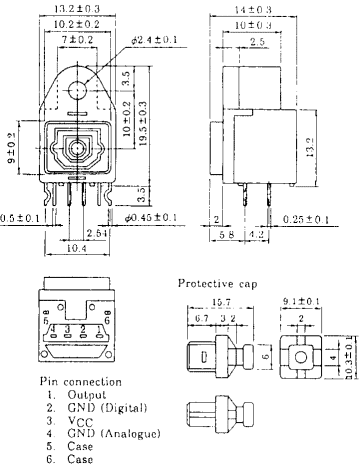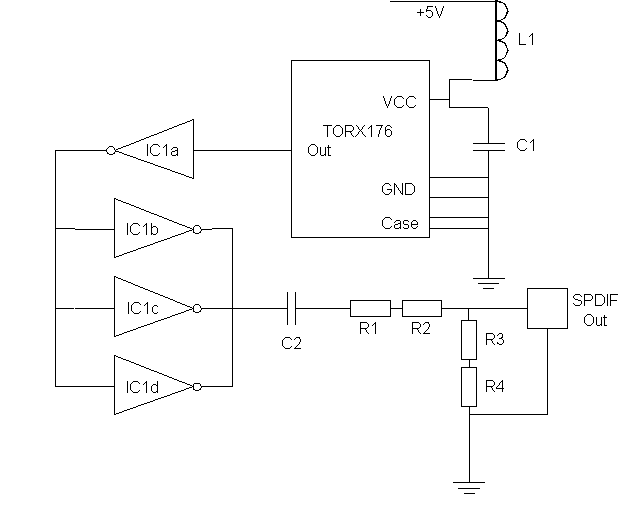# SPDIF Optical to Co-Ax Converter

## Component Information

 TORX176 Fibre Optic Receiving ModuleDatasheet Description
torx176.pdf Toshiba TORX176 Fibre Optic Receiving Module.
74hc04.pdf Texas Instruments 74HC04

## CircuitThis circuit is to be considered advance information. It has not yet been constructed or tested and therefore the design or component values may change.

 IC1 74HC04 L1 47uH C1 0.1uF C2 0.1uF R1 270R 1% Tolerance R2 68R 1% Tolerance R3 91R 1% Tolerance R4 5R6 1% Tolerance

## Circuit Description

The SPDIF Co-Ax specification is for the unloaded output to be ±0.5V with an impedance of 75R. Therefore (R3+R4) in parallel with (R1+R2) should be 75. I will call (R1+R2) R12 and (R3+R4) R34. The reason for splitting them up into two will become apparent later. The equation for this is:-

75=R12+R34/(R12*R34)

Next the output voltage of IC1 must be divided down to between 0V and 1V. The output current required to drive a loaded (75R load the other end) SPDIF output is 15mA which is 5mA per inverter. From the datasheet the inverter will output up to 4.5V at this condition. The equation for this is:-

1=4.5*R34/(R12+R34) which can be simplified to

R12=3.5*R34

Substitute this back into the first equation and you get the following resistor values:-

R12=337.5R
R34=96.4R

Now these values are not standard for resistors and using the closest values available would throw the impedance off a bit so I have used two resistors in series so that:-

R12=270+68=338 which is very close and well within the 1% tolerance of the resistors.
R34=91+5.6=96.6 which is very close and well within the 1% tolerance of the resistors.

That is the basic design. The first part of the circuit is the standard TORX176 recommended circuit. C2 is an AC coupling capacitor so the output is ±0.5V and not 0-1V. Three inverters are used to drive C2 because each one will then supply 5mA which is equivalent of a TTL load.

## Considerations

Pay close attention to the TORX176 datasheet in particular the closeness of C1 to the package and the recommendation of additional screening if the circuit is to be placed in a noisy environment such as inside a PC.

## Where To Get The Components

Quantity Order Code Description Approx cost each (UK Pounds) Supplier
1 M5R6 5R6 1% resistor 0.07 Maplin
1 M91R 91R 1% resistor 0.07 Maplin
1 M68R 68R 1% resistor 0.07 Maplin
1 M270R 270R 1% resistor 0.07 Maplin
1 UB03 SN74HC04N 0.49 Maplin
2 YR75 Minidisc 0.1uF 16V capacitor 0.19 Maplin
1 AH30 47uH inductor (not held in all stores) 0.39 Maplin
1 TORX176 TORX176 6.00 SC Electronics

Total Cost 7.54